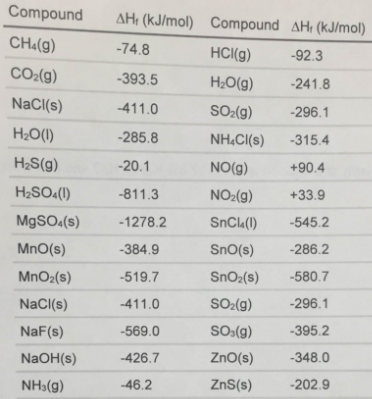# Problem: Use a standard enthalpies of formation table to determine the change in enthalpy for each of these reactions.i) NaOH(s) + HCI(g) → NaCI(s) + H 2O(g)ii) 2 CO(g) + O2(g) → 2 CO2(g)

###### FREE Expert Solution
89% (123 ratings)
###### FREE Expert Solution

ΔH°rxn = ΔH°f (products) - ΔH°f (reactants)

• check if the reaction is balanced because ΔH°f = kJ/mol

89% (123 ratings)###### Problem Details

Use a standard enthalpies of formation table to determine the change in enthalpy for each of these reactions.

i) NaOH(s) + HCI(g) → NaCI(s) + H 2O(g)

ii) 2 CO(g) + O2(g) → 2 CO2(g)What scientific concept do you need to know in order to solve this problem?

Our tutors have indicated that to solve this problem you will need to apply the Enthalpy of Formation concept. You can view video lessons to learn Enthalpy of Formation. Or if you need more Enthalpy of Formation practice, you can also practice Enthalpy of Formation practice problems.

What is the difficulty of this problem?

Our tutors rated the difficulty ofUse a standard enthalpies of formation table to determine th...as high difficulty.

How long does this problem take to solve?

Our expert Chemistry tutor, Dasha took 5 minutes and 8 seconds to solve this problem. You can follow their steps in the video explanation above.

What professor is this problem relevant for?

Based on our data, we think this problem is relevant for Professor Dixon's class at UCF.